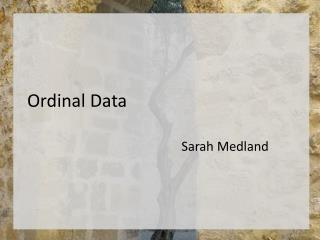DownloadDownload PresentationOrdinal Data

# Ordinal Data

Download Presentation## Ordinal Data

- - - - - - - - - - - - - - - - - - - - - - - - - - - E N D - - - - - - - - - - - - - - - - - - - - - - - - - - -
##### Presentation Transcript

1. Ordinal Data Sarah Medland

2. A plug for OpenMx? • Very few packages can handle ordinal data adequately… • OpenMx can also be used for more than just genetic analyses • Regression • Polycohoric correlations • Factor analysis...

3. Two approaches to the liability threshold model • Problem • Ordinal data has 1 less degree of freedom • MZcov, DZcov, Prevalence • No information on the variance • Thinking about our ACE/ADE model • 4 parameters being estimated • A C E mean • ACE/ADE model is unidentified without adding a constraint

4. Two approaches to the liability threshold model • Solution? • Traditional • Maps data to a standard normal distribution • Total variance constrained to be 1 • Alternate • Fixes an alternate parameter (usually E) • Estimates the remaining parameters

5. Traditional Approach • Imagine we have a set of binary data • Trait – lifetime cannabis use • Never Smoked/Ever Smoked

6. Twin 1 cannabis use • 0 = never used

7. Twin 1 cannabis use

8. Twin 1 cannabis use Liability or ‘risk’ of initiation distribution Just because an individual has never used cannabis does not mean their ‘risk’ of initiation is zero

9. Mean = .47 SD =.499 Non Smokers =53% The observed phenotype is an imperfect measurement of an underlying continuous distribution ie Obesity vs BMI MDD vs quantitative depression scales

10. Raw data distribution Mean = .47 SD =.499 Non Smokers =53% Threshold =.53 Standard normal distribution Mean = 0 SD =1 Non Smokers =53% Threshold =.074

11. Threshold = .074 – Huh what? • How can I work this out • Excell • =NORMSINV() • Thresholds.xls

12. Why rescale the data this way? • Convenience • Variance always 1 • Mean is always 0 • We can interpret the area under a curve between two z-values as a probability or percentage

13. Threshold.R

14. Threshold.R

15. Threshold = .075 – Huh what?

16. What about more than 2 categories? • Very similar • We create a matrix containing the 1st threshold and the displacements between subsequent matrices • We then add the 1st threshold and the displacement to obtain the subsequent thresholds

17. 2.2 -1 1.2 3 -3 0 Mx Threshold Specification: 3+ Cat. Threshold matrix: T Full 2 2 Free Twin 1 Twin 2 1st threshold increment

18. 2.2 -1 1.2 3 -3 0 Mx Threshold Specification: 3+ Cat. Threshold matrix: T Full 2 2 Free Twin 1 Twin 2 1st threshold increment MxAlgebraL%*%T

19. 2.2 -1 1.2 3 -3 0 Mx Threshold Specification: 3+ Cat. Threshold matrix: T Full 2 2 Free Twin 1 Twin 2 1st threshold increment MxAlgebraL%*%T 2nd threshold

20. Check the xlsspreadsheet…

21. Two approaches to the liability threshold model • Solution? • Traditional • Maps data to a standard normal distribution • Total variance constrained to be 1 • Alternate • Fixes an alternate parameter • Binary or Ordinal data fix E • Ordinal data fix 1st two thresholds (aka invariant threshold approach) • Estimate the remaining parameters

22. Models are equivalent, but… • Alternate approach means the data is no longer mapped to a standard normal • No easy conversion to % • Makes it difficult to compare between groups as the scaling is now arbitrary

23. We are going to run traditional and FixedE ACE models with binary data • twinAceBin-Traditional.R • twinAceBin-FixE.R • There is are scripts in the folder that have multiple threshold models in them – take a look later • twinAceOrd-Traditional.R • twinAceOrd-FixE.R • twinAceOrd-FixThresholds.R

24. Lisbon Castle